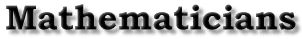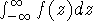| Cauchy's Life | Cauchy's Mathematical Accomplishments | Cauchy's Criterion for Convergence | Bibliography | Back to the front page Cauchy's Mathematical Accomplishments Somsack Chaitesipaseut Cauchy had many mathematical accomplishments. It would take several pages, if not several volumes of books, to cover everything Cauchy has done or has had an impact on in the field of math and science. Several areas where he made his presence known are complex functions, optics, elasticity, group theory, mathematical physics and astronomy, hydrodynamics, and differential equations [Freudenthal, p. 135]. In calculus, Cauchy made his presence known by formulating conditions and proving propositions such as the Cauchy criterion for convergence, that a continuous function has a zero between the endpoints where its signs are different, and ``invented what is now called the Jacobian,'' which he restricted to two and three dimensions [Freudenthal, p. 136]. Cauchy's Cours d'Analyse de l'École Royale Polytechnique of 1821 has a major impact in today's understanding of limits, continuity, and integrals [Katz, p. 707-708]. In fact, some say that Cauchy gave the first reasonably successful rigorous foundation for calculus [Grabiner, p. viii]. Then there are those who feel that Cauchy had an even greater impact in the field of complex functions. It was in his Cours d'Analyse of 1821 that we could find the ``first comprehensive theory of complex numbers'' [Freudenthal, p. 137]. In it, Cauchy justifies algebraic and limit operations on complex numbers, incorporates absolute values, and defines continuity for complex functions. Cauchy also used logarithmic residues as a means in defining the number of roots of a function in a domain, as well as the formula for the sums over the roots of the function. And then there are those who believe that ``Cauchy's most important result here [in the field of complex functions] is'' the computation of[Freudenthal, p. 137-138]. Cauchy was not a slacker in the field of algebra as well. He contributed to algebra by working on the inverse of a matrix, by formulating theorems on determinants formed by subdeterminants, and proved that if a polynomial assumes more than two values under permutations of its n variables, then it assumes at least p values, where p is the largest prime in n (which is a generalization of one of Ruffini's theorems) [Freudenthal, p. 142]. Plus, algebra is another field where Cauchy has a theorem named after him, ``Cauchy's theorem'' for finite groups. The theorem says, ``For any prime p dividing the order there is an element of order p.'' | Cauchy's Life | Cauchy's Mathematical Accomplishments | Cauchy's Criterion for Convergence | Bibliography | Back to the front page